# How to get or reference cell from another worksheet in Excel?

Referencing a cell in Excel from another sheet means that the user is indirectly using the value or formula in a cell from a different sheet within the same workbook. Let’s understand some common benefits of using reference cells from another worksheet in Excel. It helps in improving the organization of data. It allows the user to separate the data and calculations into different sheets, making it easier to organize and manage the workbook. Another common benefit is increased efficiency.

Referencing cells in another sheet allows users to reuse formulas and data without any need to copy and paste the same data to multiple sheets. This can save time and reduce the risk of errors. It also increases data accuracy. While using reference cells in another sheet, the user is 100% sure that the data value is correct and can generate valid outcomes only. This can help prevent errors that can occur when copying and pasting data between sheets. Referenced cells make data collaboration easy.

This simply means that multiple people can use the referencing cells in another sheet at the same time. Same without accidentally changing or deleting data in other sheets. Last but not least it allows users to analyze data efficiently as it allows users to perform more complex analyses and calculations, such as using data from different sheets to create pivot tables or charts. This article contains two easy examples to guide users about the process of using the reference data.

## Example 1: To understand the process of accessing reference variable from another sheet to current sheet:

### Step 1

For this example, will consider two sheets, that is sheets A and B. On sheet A, create a table with two rows in column C. This column contains some data values.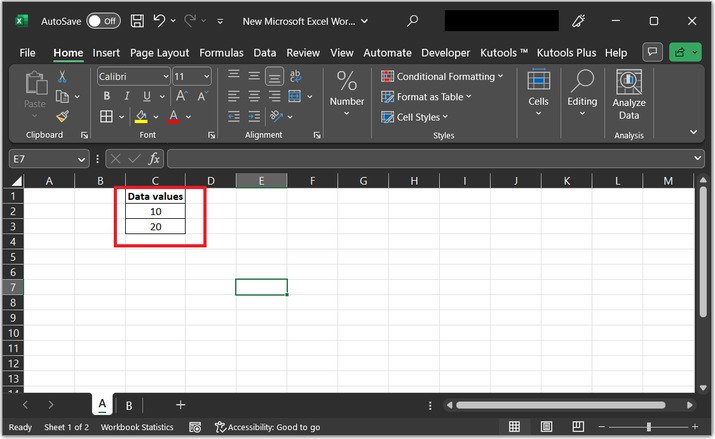### Step 2

Before, moving towards the further steps, consider the referenced cell in another sheet, user needs to specify the sheet name with an exclamation point (!), then write the reference of the cell. For instance, to reference cell A1 on Sheet2 from Sheet1, the user would enter "=Sheet2!A1" in the formula bar.

In this example will copy the value of the C2 cell from sheet A to Sheet B.

So, the required formula syntax will be: sheet_name ! column_name cell_value.

For this example, type the formula “=A!C2” on the B Excel sheet. For more clarification refer to the below given snapshot −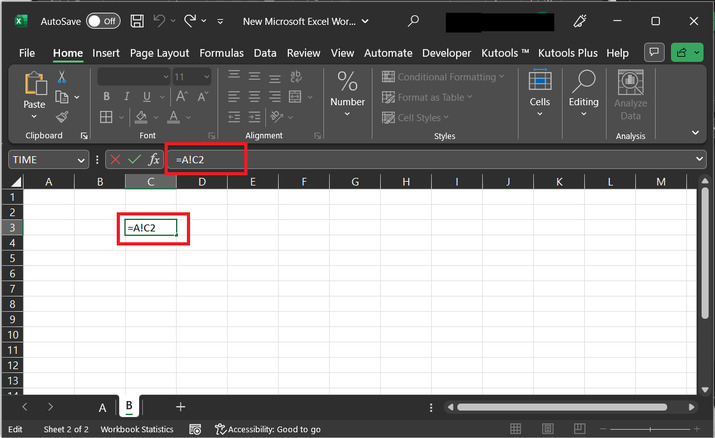### Step 3

Press the “Enter” key and the required result value that is 10, is displayed on the Excel sheet, as given below −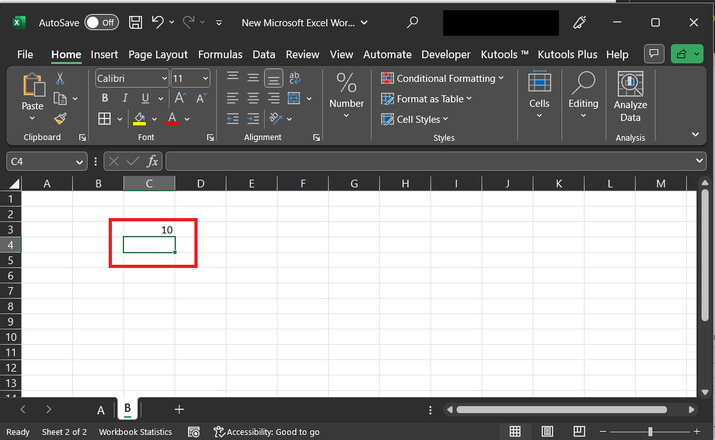## Example 2: To understand the process of using the sum formula by accessing the reference variable from another sheet to the current:

### Step 1

For this example, will consider two sheets, that is sheets A and B. On sheet A, create a table with two rows in column C. This column contains some data values.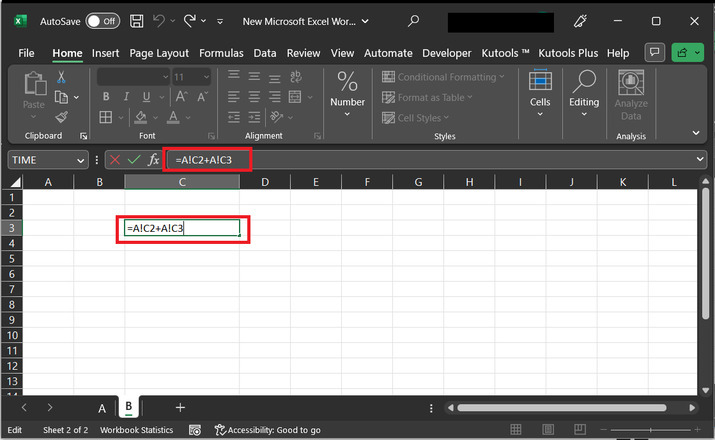### Step 2

In this example, the user wants to add the sum of two values provided in the above Excel sheet. In more simple words, this example wants the user to add C2 and C3 cell data.

### The syntax for the required formula:

Sheet_name!column_name1 cell_range + Sheet_name!column_name2 cell_range

### This same can be achieved by below given two methods:

1) Use formula "=SUM(A!C2, A!C3)" in the formula bar.

2) Use formula “=A!C2+A!C3”.

Please note that the provided formula will perform the same task. But here will demonstrate the use of the second formula.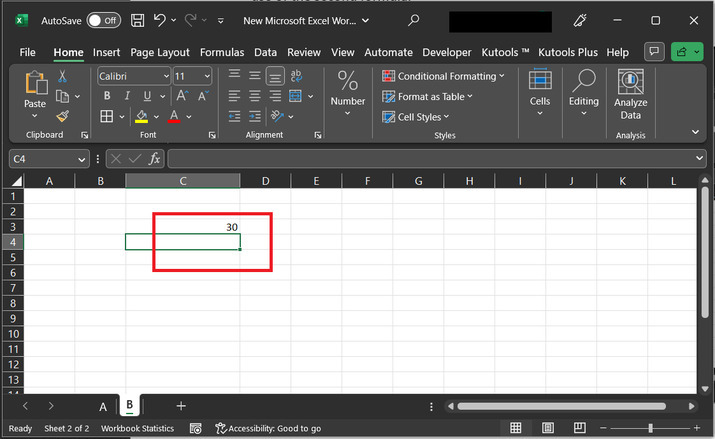### Step 3:

Press the “Enter” key and the required result value that is 30, is displayed on the Excel sheet, as given below −

## Conclusion

The provided two examples demonstrate to the user the way to access the referenced cell from another sheet to the current sheet. In the first example, the reference of the previous sheet is given along with the specified cell value. The second example will guide the way to process the data by using a simple sum formula. All the guided steps are detailed and explained.